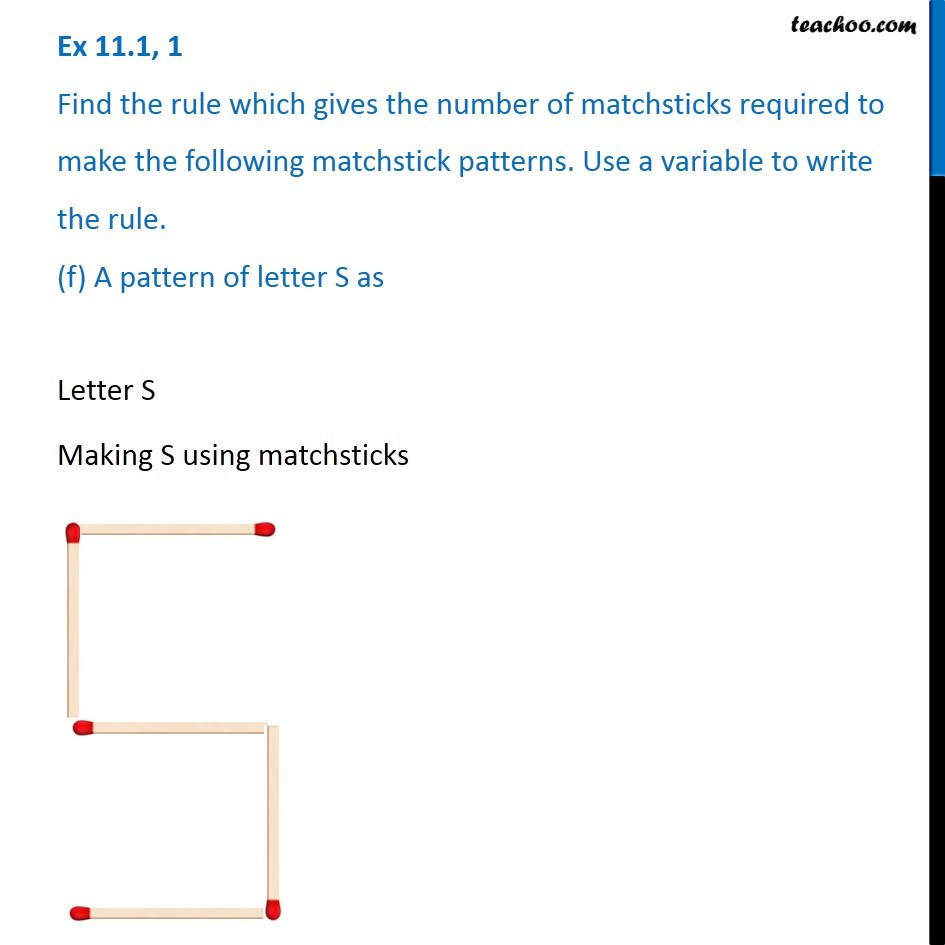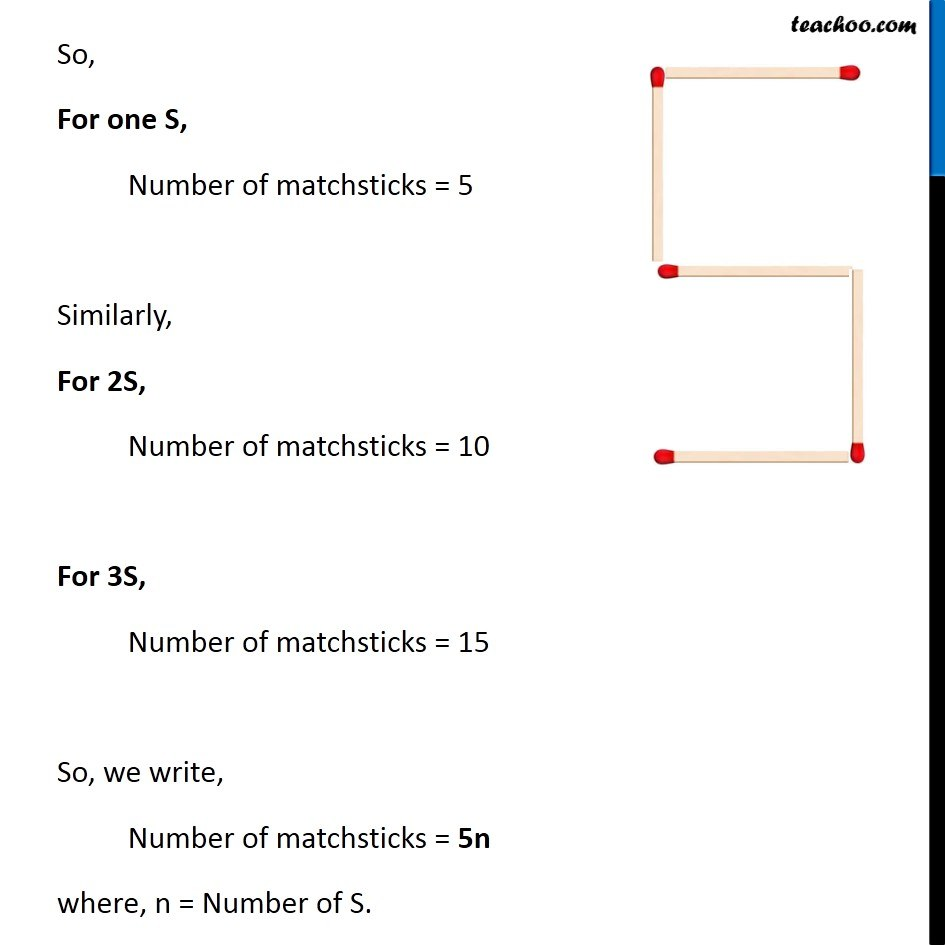Ex 11.1

Chapter 11 Class 6 Algebra
Serial order wiseLearn in your speed, with individual attention - Teachoo Maths 1-on-1 Class

### Transcript

Ex 11.1, 1 Find the rule which gives the number of matchsticks required to make the following matchstick patterns. Use a variable to write the rule. (f) A pattern of letter S asEx 11.1, 1 Find the rule which gives the number of matchsticks required to make the following matchstick patterns. Use a variable to write the rule. (f) A pattern of letter S asSo, For one S, Number of matchsticks = 5 Similarly, For 2S, Number of matchsticks = 10 For 3S, Number of matchsticks = 15 So, we write, Number of matchsticks = 5n where, n = Number of S.